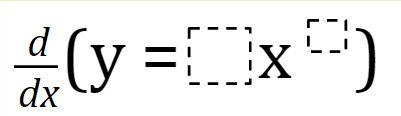# Derivatives – Power Rule

Directions: Using the digits 1 to 9 at most one time each, fill in the boxes to create a function such that at x = 2, the derivative (at that point) would fall in the interval of {0, 48}### Hint

One might consider solving the equation at the extremes and means of the interval of solutions